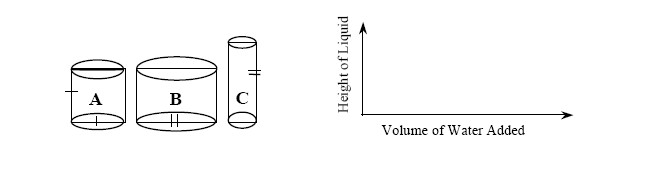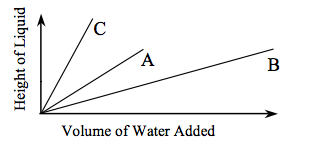### Home > A2C > Chapter 1 > Lesson 1.2.3 > Problem1-111

1-111.

Imagine that you add water to the beakers shown below (labeled $A, B,\text{ and }C$). Sketch a graph for each beaker to show the relationship between the volume of water added and the height of the water in each beaker. Put all three graphs on one set of axes (you may want to use colored pencils to distinguish the graphs). What are the independent and dependent variables?Independent Variable: a variable whose value determines the value of other variables.
Dependent Variable: a variable whose value is determined by the value of an independent variable.

Independent Variable: volume of water
Dependent Variable: height of liquid